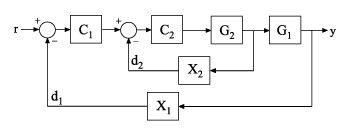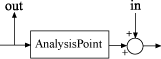# getIOTransfer

Closed-loop transfer function from generalized model of control system

## Syntax

``H = getIOTransfer(T,in,out)``
``H = getIOTransfer(T,in,out,openings)``

## Description

example

````H = getIOTransfer(T,in,out)` returns the transfer function from specified inputs to specified outputs of a control system, computed from a closed-loop generalized model of the control system.```

example

````H = getIOTransfer(T,in,out,openings)` returns the transfer function calculated with one or more loops open.```

## Examples

collapse all

Analyze responses of a control system by using `getIOTransfer` to compute responses between various inputs and outputs of a closed-loop model of the system.

Consider the following control system.Create a `genss` model of the system by specifying and connecting the numeric plant models `G1` and `G2`, the tunable controllers `C1` and `C2`, and the `AnalysisPoint` blocks `X1` and `X2` that mark potential loop-opening or signal injection sites.

```G1 = tf(10,[1 10]); G2 = tf([1 2],[1 0.2 10]); C1 = tunablePID('C','pi'); C2 = tunableGain('G',1); X1 = AnalysisPoint('X1'); X2 = AnalysisPoint('X2'); T = feedback(G1*feedback(G2*C2,X2)*C1,X1); T.InputName = 'r'; T.OutputName = 'y';```

If you tuned the free parameters of this model (for example, using the tuning command `systune`), you might want to analyze the tuned system performance by examining various system responses.

For example, examine the response at the output, y, to a disturbance injected at the point ${d}_{1}$.

`H1 = getIOTransfer(T,'X1','y');`

`H1` represents the closed-loop response of the control system to a disturbance injected at the implicit input associated with the `AnalysisPoint` block `X1`, which is the location of ${d}_{1}$:`H1` is a `genss` model that includes the tunable blocks of `T`. If you have tuned the free parameters of `T`, `H1` allows you to validate the disturbance response of your tuned system. For example, you can use analysis commands such as `bodeplot` or `stepplot` to examine the responses of `H1`. You can also use `getValue` to obtain the current value of `H1`, in which all the tunable blocks are evaluated to their current numeric values.

Similarly, examine the response at the output to a disturbance injected at the point ${d}_{2}$.

`H2 = getIOTransfer(T,'X2','y');`

You can also generate a two-input, one-output model representing the response of the control system to simultaneous disturbances at both ${d}_{1}$ and ${d}_{2}$. To do so, provide `getIOTransfer` with a cell array that specifies the multiple input locations.

`H = getIOTransfer(T,{'X1','X2'},'y');`

Compute the response from r to y of the following cascaded control system, with the inner loop open, and the outer loop closed.Create a `genss` model of the system by specifying and connecting the numeric plant models `G1` and `G2`, the tunable controllers `C1` and `C2`, and the `AnalysisPoint` blocks `X1` and `X2` that mark potential loop-opening or signal injection sites.

```G1 = tf(10,[1 10]); G2 = tf([1 2],[1 0.2 10]); C1 = tunablePID('C','pi'); C2 = tunableGain('G',1); X1 = AnalysisPoint('X1'); X2 = AnalysisPoint('X2'); T = feedback(G1*feedback(G2*C2,X2)*C1,X1); T.InputName = 'r'; T.OutputName = 'y';```

If you tuned the free parameters of this model (for example, using the tuning command `systune`), you might want to analyze the tuned system performance by examining various system responses.

For example, compute the response of the system with the inner loop open, and the outer loop closed.

`H = getIOTransfer(T,'r','y','X2');`

By default, the loops are closed at the analysis points `X1` and `X2`. Specifying `'X2'` for the `openings` argument causes `getIOTransfer` to open the loop at `X2` for the purposes of computing the requested transfer from r to y. The switch at `X1` remains closed for this computation.

## Input Arguments

collapse all

Model of a control system, specified as a generalized state-space model (`genss`).

Input to extracted transfer function, specified as a character vector or cell array of character vectors. To extract a multiple-input transfer function from the control system, use a cell array of character vectors. Each specified input must match either:

• An input of the control system model `T`; that is, a channel name from`T.InputName`.

• An analysis point in `T`, corresponding to a channel of an `AnalysisPoint` block in `T`. To get the list of available analysis points in `T`, use `getPoints(T)`.

When you specify an analysis point as an input `in`, `getIOTransfer` uses the input implicitly associated with the `AnalysisPoint` channel, arranged as follows.This input signal models a disturbance entering at the output of the switch.

If an analysis point has the same name as an input of `T`, then `getIOTransfer` uses the input of `T`.

Example: `{'r','X1'}`

Output of extracted transfer function, specified as a character vector or cell array of character vectors. To extract a multiple-output transfer function from the control system, use a cell array of character vectors. Each specified output must match either:

• An output of the control system model `T`; that is, a channel name from `T.OutputName`.

• An analysis point in `T`, corresponding to a channel of an `AnalysisPoint` block in `T`. To get the list of available analysis points in `T`, use `getPoints(T)`.

When you specify an analysis point as an output `out`, `getIOTransfer` uses the output implicitly associated with the `AnalysisPoint` channel, arranged as follows.If an analysis point has the same name as an output of `T`, then `getIOTransfer` uses the output of `T`.

Example: `{'y','X2'}`

Locations for opening feedback loops for computation of the response from `in` to `out`, specified as a character vector or cell array of character vectors that identify analysis points in `T`. Analysis points are marked by `AnalysisPoint` blocks in `T`. To get the list of available analysis points in `T`, use `getPoints(T)`.

Use `openings` when you want to compute the response from `in` to `out` with some loops in the control system open. For example, in a cascaded loop configuration, you can calculate the response from the system input to the system output with the inner loop open.

## Output Arguments

collapse all

Closed-loop transfer function of the control system `T` from `in` to `out`, returned as a generalized state-space model (`genss`).

• If both `in` and `out` specify a single signal, then `T` is a SISO `genss` model.

• If `in` or `out` specifies multiple signals, then `T` is a MIMO `genss` model.

## Tips

• You can use `getIOTransfer` to extract various subsystem responses, given a generalized model of the overall control system. This is useful for validating responses of a control system that you tune with tuning commands such as `systune`.

For example, in addition to evaluating the overall response of a tuned control system from inputs to outputs, you can use `getIOTransfer` to extract the transfer function from a disturbance input to a system output. Evaluate the responses of that transfer function (such as with `step` or `bode`) to confirm that the tuned system meets your disturbance rejection requirements.

• `getIOTransfer` is the `genss` equivalent to the Simulink® Control Design™ `getIOTransfer` command, which works with the `slTuner` and `slLinearizer` interfaces. Use the Simulink Control Design command when your control system is modeled in Simulink.